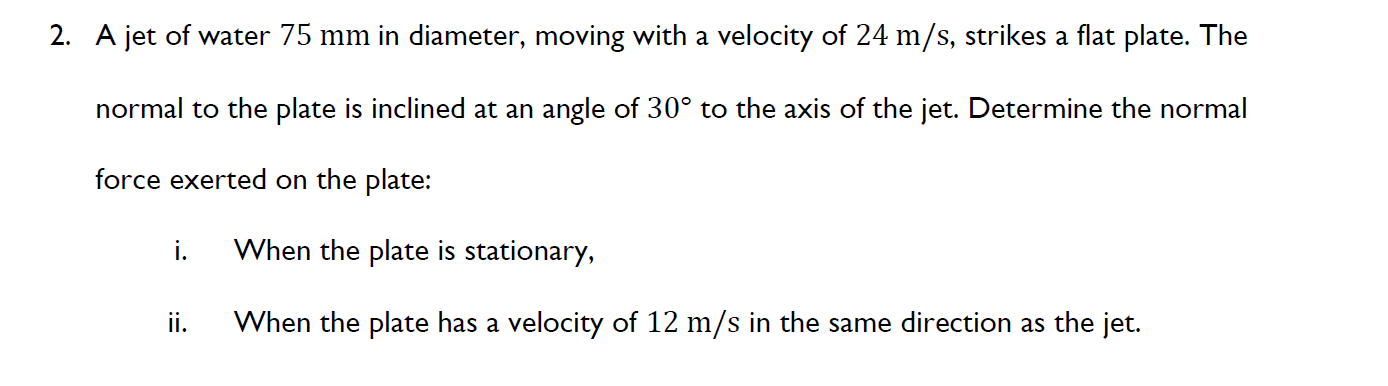# A jet of water 75 mm in diameter, moving with a velocity of 24 m/s, strikes a flat plate. The normal to the plate is inclined at an angle of 30° to the axis of the jet. Determine the normal force exerted on the plate: i. When the plate is stationary, ii. When the plate has a velocity of 12 m/s in the same direction as the jet.

Question-AnswerCategory: Fluid MechanicsA jet of water 75 mm in diameter, moving with a velocity of 24 m/s, strikes a flat plate. The normal to the plate is inclined at an angle of 30° to the axis of the jet. Determine the normal force exerted on the plate: i. When the plate is stationary, ii. When the plate has a velocity of 12 m/s in the same direction as the jet.

A jet of water 75 mm in diameter, moving with a velocity of 24 m/s, strikes a flat plate. The normal to the plate is inclined at an angle of 30° to the axis of the jet. Determine the normal force exerted on the plate: i. When the plate is stationary, ii. When the plate has a velocity of 12 m/s in the same direction as the jet.